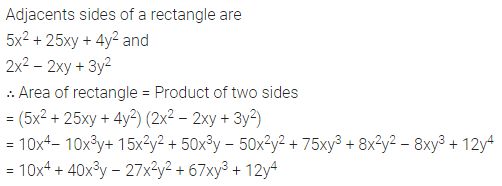## ML Aggarwal Class 8 Solutions for ICSE Maths Chapter 10 Algebraic Expressions and Identities Ex 10.3

Question 1.
Multiply:
(i) (5x – 2) by (3x + 4)
(ii) (ax + b) by (cx + d)
(iii) (4p – 7) by (2 – 3p)
(iv) (2x2 + 3) by (3x – 5)
(v) (1.5a – 2.5b) by (1.5a + 2.56)
(vi) $$\left(\frac{3}{7} p^{2}+4 q^{2}\right) \text { by } 7\left(p^{2}-\frac{3}{4} q^{2}\right)$$
Solution: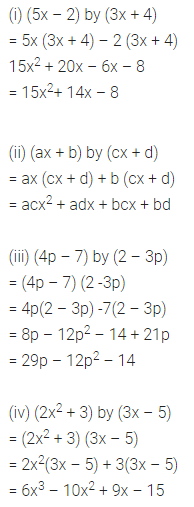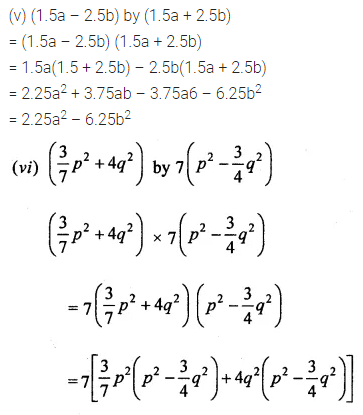Question 2.
Multiply:
(i) (x – 2y + 3) by (x + 2y)
(ii) (3 – 5x + 2 × 2) by (4x – 5)
Solution: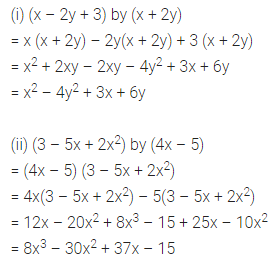Question 3.
Multiply:
(i) (3x2 – 2x – 1) by (2x2 + x – 5)
(ii) (2 – 3y – 5y2) by (2y – 1 + 3y2)
Solution:Question 4.
Simplify:
(i) (x2 + 3) (x – 3) + 9
(ii) (x + 3) (x – 3) (x + 4) (x – 4)
(iii) (x + 5) (x + 6) (x + 7)
(iv) (p + q – 2r) (2p – q + r) – 4qr
(v) (p + q) (r + s) + (p – q)(r – s) – 2(pr + qs)
(vi) (x + y + z) (x – y + z) + (x + y – z) (-x + y + z) – 4zx
Solution: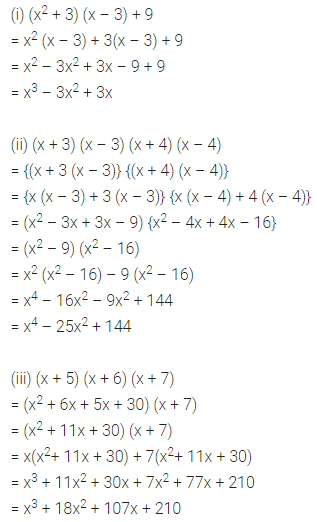Question 5.
If two adjacent sides of a rectangle are 5x2 + 25xy + 4y2 and 2x2 – 2xy + 3y2, find its area.
Solution: